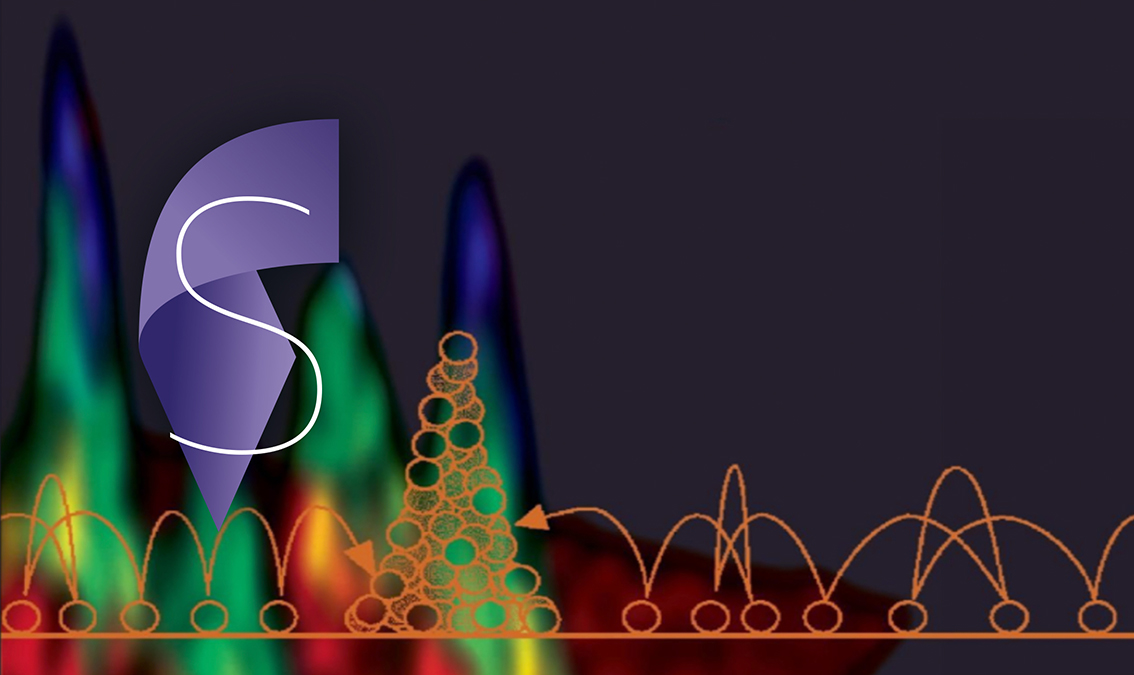EPFLWe explore statistical physics in both classical and open quantum systems. Additionally, we will cover probabilistic data analysis that is extremely useful in many applications.

This course covers non-equilibrium statistical processes and the treatment of fluctuation dissipation relations by Einstein, Boltzmann and Kubo. Moreover, the fundamentals of Markov processes, stochastic differential and Fokker Planck equations, mesoscopic master equation, etc will be treated in detail. Prior knowledge of statistical physics is highly recommended but not required.

### What you'll learn

• Formulate statistical processes mathematically
• Solve the quantum master equation using QuTip in Python
• Apply numerical simulation tools to non-equilibrium systems
• Explore the quantum optical numerical Toolbox (MATLAB)
• Visualize non-equilibrium processes numerically using Jupyter Notebooks
• Elaborate modern examples from Literature of Non-Equilibrium Processes
• Apply EMCEE Python package to Bayesian statistical data analysis

### Instructor

• Prof. Tobias J. Kippenberg

1. Course Number

StatPhys
2. Classes Start

3. Classes End

4. Estimated Effort

3-5 hours / week
Enroll